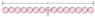# Rotational Inertia

Riman643
Homework Statement:
The figure shows an arrangement of 15 identical disks that have been glued together in a rod-like shape of length L = 1.6900 m and (total) mass M = 104.0 mg. The arrangement can rotate about a perpendicular axis through its central disk at point O. (a) What is the rotational inertia of the arrangement about that axis? Give your answer to four significant figures. (b) If we approximated the arrangement as being a uniform rod of mass M and length L, what percentage error would we make in using the formula in Table 10-2e to calculate the rotational inertia?
Relevant Equations:
I = 1/12 ML^2
I = 1/2 mr^2
This question is ridiculously complex for me. I know how to find the Inertia if it was a rod

##I =\frac{1}{12} ML^{2} = \frac{1}{12}(0.000104)(1.6900)^2 =0.00002475 kg * m^2##

To find the Inertia for the individual disks I am having trouble. Not sure how to add them all up together. Guidance would be greatly appreciated.

#### Attachments

•fig10_38.gif
3.5 KB · Views: 206

Homework Helper
Gold Member
2022 Award
To find the Inertia for the individual disks I am having trouble
Consider the nth disk from O, to the right, say.
What is its MoI about its own centre?
How far is its centre from O?
Using the parallel axis theorem, what is its MoI about O?

Riman643
I think I get where you are going. Every disk will be:

##I = \frac{1}{2} mr^{2} = \frac{1}{2} (\frac{0.000104}{15})(\frac{1.6900}{\frac{15}{2}})^{2} = 0.000000011 kg * m^2##

Then using the parallel axis theorem it would be:
##I = \frac{1}{2}mr^{2} + md^{2}##

but which disk do I use for the ##md^{2}## part? And would the distance to let's say the one to the right and left of the center bed twice the radius?

Last edited:
Homework Helper
Gold Member
2022 Award
Every disk will be
How many radii make up length L?
would the distance to let's say the one to the right and left of the center bed twice the radius?
Yes. What about the next ones along?

Riman643
How many radii make up length L?

15? Would that then make the parallel axis theorem in this particular case:

##I_{O} = 15(\frac{1}{2}mr^{2}) + md^{2}##
Yes. What about the next ones along?

The next ones along would be 4 times the length of the radius. So I should use the furthest one away? 14 times the length of the radius?

Homework Helper
Gold Member
2022 Award
15?
No, try again.
The next ones along would be 4 times the length of the radius.
Yes.
So I should use the furthest one away?
No, each makes the contribution it makes. Sum the series.

Riman643
No, try again.
Oops. It's 30 that will make up the entire length

No, each makes the contribution it makes. Sum the series.
So would a correct formula with a series of distances be:

##I_{O} = 15mr^{2} + 2mr^{2}(2 + 4 + 6 + 8 +10 +12 + 14)##

Homework Helper
Gold Member
2022 Award
Oops. It's 30 that will make up the entire length

So would a correct formula with a series of distances be:

##I_{O} = 15mr^{2} + 2mr^{2}(2 + 4 + 6 + 8 +10 +12 + 14)##
Close...
There's something you left out on the 2, 4, 6 etc.

Riman643
Close...
There's something you left out on the 2, 4, 6 etc.
Oops. took out the square when I took out ##r^{2}##. I got the answer right for the first part. But for some reason I am struggling getting the percentage with the rod wrong. For the rod I get ##24.75 mg * m^{2}## and for the actual Inertia I get ##25.02 mg * m^{2}##. Then I take ##(1 - \frac{24.75}{25.02}) * 100 = 1.067 %##. Not sure what I am doing wrong with that.